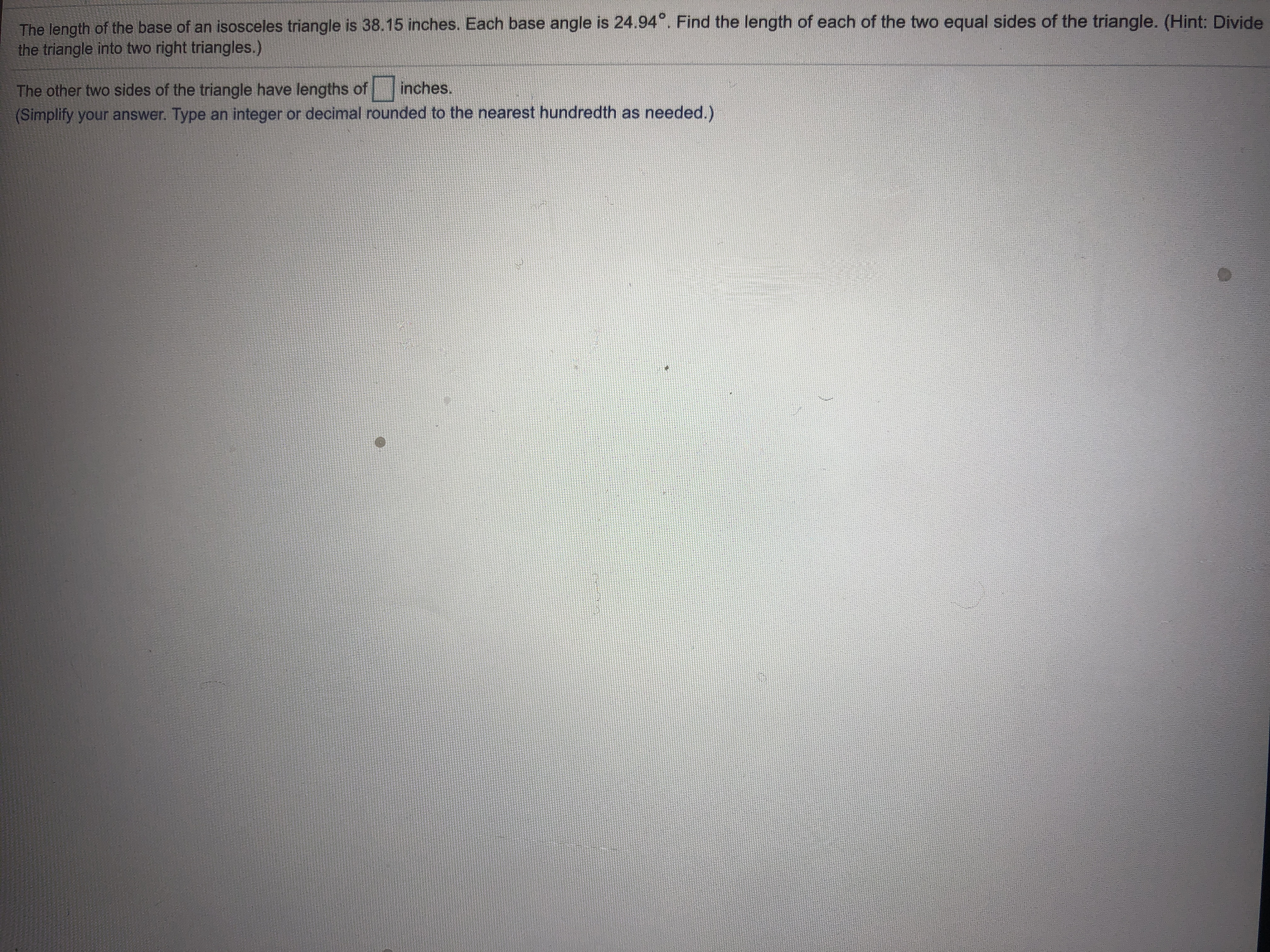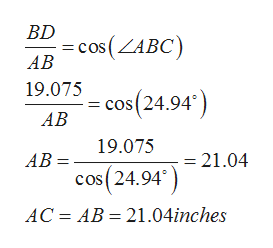# The length of the base of an isosceles triangle is 38.15 inches. Each base angle is 24.94. Find the length of each of the two equal sides of the triangle. (Hint: Dividethe triangle into two right triangles.)The other two sides of the triangle have lengths of(Simplify your answer. Type an integer or decimal rounded to the nearest hundredth as needed.)inches.

Question
3 viewshelp_outlineImage TranscriptioncloseThe length of the base of an isosceles triangle is 38.15 inches. Each base angle is 24.94. Find the length of each of the two equal sides of the triangle. (Hint: Divide the triangle into two right triangles.) The other two sides of the triangle have lengths of (Simplify your answer. Type an integer or decimal rounded to the nearest hundredth as needed.) inches. fullscreen
check_circle

Step 1

Let ABC be the isosceles triangle with base BC and equal sides AB and AC. Then BC=38.15 inches and angle ABC=angle ACB=24.94 degrees. Let AD be the perpendicular drawn from a to side BC . Since triangles ABD and ACD are congruent , so BD=DC, that is BD=BC/2=19.075 inches

Step 2

In triangle ABD ...help_outlineImage TranscriptioncloseBD cos(ZABC) АВ 19.075 cos(24.94 АВ 19.075 21.04 АВ : cos(24.94 AC AB 21.04inches fullscreen

### Want to see the full answer?

See Solution

#### Want to see this answer and more?

Solutions are written by subject experts who are available 24/7. Questions are typically answered within 1 hour.*

See Solution
*Response times may vary by subject and question.
Tagged in

### Trigonometric Ratios